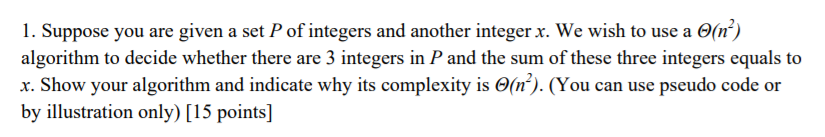# Question & Answer: Suppose you are given a set P of integers and another integer x. We wish t…..Don't use plagiarized sources. Get Your Custom Essay on
Question & Answer: Suppose you are given a set P of integers and another integer x. We wish t…..
GET AN ESSAY WRITTEN FOR YOU FROM AS LOW AS \$13/PAGE

Suppose you are given a set P of integers and another integer x. We wish to use a Theta(n^2) algorithm to decide whether there are 3 integers in P and the sum of these three integers equals to x. Show your algorithm and indicate why its complexity is Theta(n^2). (You can use pseudo code or by illustration only)

Algorithm to find three numbers from an array of numbers so that its sum is x

First step will be to sort the numbers with any algorithm (Quicksort or mergesort with nlogn complexity). Once sorted we can apply following way to find out the the three numbersL

1.Place a pointer at the start of the array.(Say i)
2.Place a pointer at the last of the array (Say j)
3.Place a pointer just after i (Say k)

Now for every i, perform a[i] + a[j] + a[k] .There can be three posibilities:

1.Sum is equal to x : answer is found
2.Sum is more than x : reduce j by 1
3.Sum is less than x : increase k by 1

At the end k mand j cross each other and we know that we exhausted all the possibilities. So for every i we will have maximum of n movements. So we have to do this for every i till we exhaust all the elements. So for n elements in the array we have to have n^2 comparisons or movements.It means the complexity will be O(n^2)